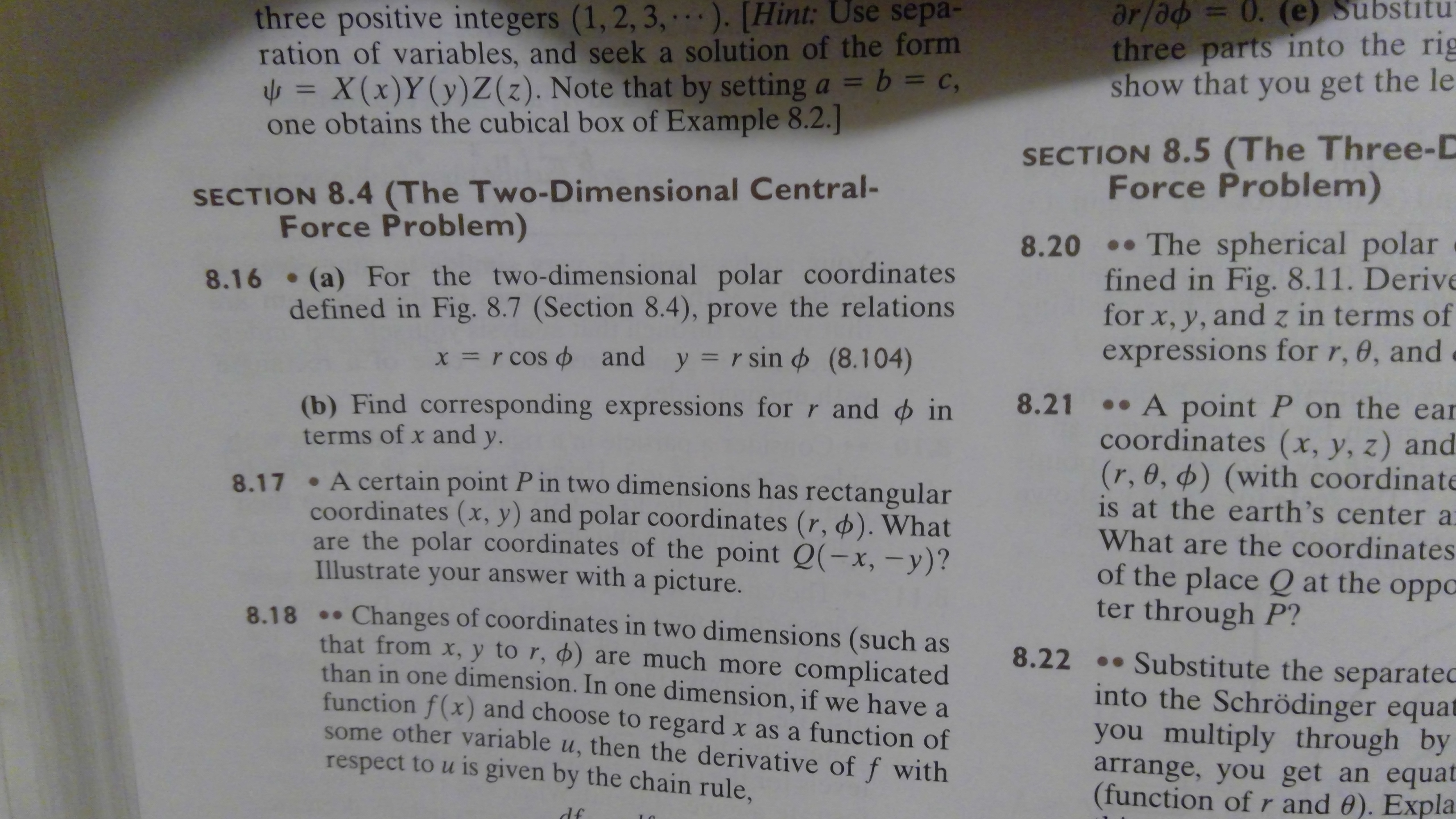# ar/ad= 0. (e) Substituthree parts into the rigshow that you get the lethree positive inte gers (1, 2, 3, . ). [Hint: Use sepa-ration of variables, and seek a solution of the form= X(x) Y (y) Z (z). Note that by setting a b = cobtains the cubical box of Example 8.2.]SECTION 8.5 (The Three-DForce Problem)SECTION 8.4 (The Two-Dimensional Central-Force Problem)8.20 The spherical polarfined in Fig. 8.11. Derivefor x, y, and z in terms ofexpressions for r, 0, and8.16 (a) For the two-dimensional polar coordinatesdefined in Fig. 8.7 (Section 8.4), prove the relationsX = r cos and y r sin (8.104)8.21 A point P on the earcoordinates (x, y, z) and(r, 0, ø) (with coordinateis at the earth's center aWhat are the coordinatesof the place Q at the oppoCter through P?(b) Find corresponding expressions for r and interms of x andу.A certain point P in two dimensions has rectangularcoordinates (x, y) and polar coordinates (r, ). Whatare the polar coordinates of the point Q(-x, -y)?Illustrate your answer with a picture.8.17Changes of coordinates in two dimensions (such asthat from x, y to r, ) are much more complicatedthan in one dimension. In one dimension, if we have afunction f(x) and choose to regard x as a function ofsome other variable u, then the derivative of f withrespect to u is given by the chain rule,8.188.22.Substitute the separatecinto the Schrödinger equatyou multiply through byarrange, you get an equat(function of r and 0). Expladf

Question
3 views

For Problem 8.16, how do I prove the relations and give the correct expressions?help_outlineImage Transcriptionclosear/ad= 0. (e) Substitu three parts into the rig show that you get the le three positive inte gers (1, 2, 3, . ). [Hint: Use sepa- ration of variables, and seek a solution of the form = X(x) Y (y) Z (z). Note that by setting a b = c obtains the cubical box of Example 8.2.] SECTION 8.5 (The Three-D Force Problem) SECTION 8.4 (The Two-Dimensional Central- Force Problem) 8.20 The spherical polar fined in Fig. 8.11. Derive for x, y, and z in terms of expressions for r, 0, and 8.16 (a) For the two-dimensional polar coordinates defined in Fig. 8.7 (Section 8.4), prove the relations X = r cos and y r sin (8.104) 8.21 A point P on the ear coordinates (x, y, z) and (r, 0, ø) (with coordinate is at the earth's center a What are the coordinates of the place Q at the oppoC ter through P? (b) Find corresponding expressions for r and in terms of x and у. A certain point P in two dimensions has rectangular coordinates (x, y) and polar coordinates (r, ). What are the polar coordinates of the point Q(-x, -y)? Illustrate your answer with a picture. 8.17 Changes of coordinates in two dimensions (such as that from x, y to r, ) are much more complicated than in one dimension. In one dimension, if we have a function f(x) and choose to regard x as a function of some other variable u, then the derivative of f with respect to u is given by the chain rule, 8.18 8.22.Substitute the separatec into the Schrödinger equat you multiply through by arrange, you get an equat (function of r and 0). Expla df fullscreen
check_circle

Step 1

Draw a figure to represent a point using both polar and Cartesian coordinates.

Step 2

(a)

Consider the right angled triangle OMP.

Write an expression for cosϕ using trigonometry

Step 3

Write an expression for sinϕ u...

### Want to see the full answer?

See Solution

#### Want to see this answer and more?

Solutions are written by subject experts who are available 24/7. Questions are typically answered within 1 hour.*

See Solution
*Response times may vary by subject and question.
Tagged in

### Science